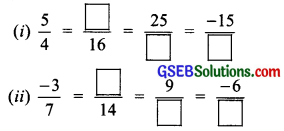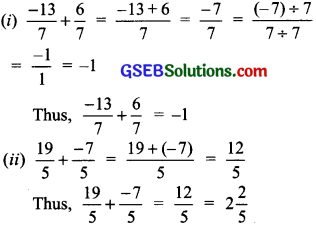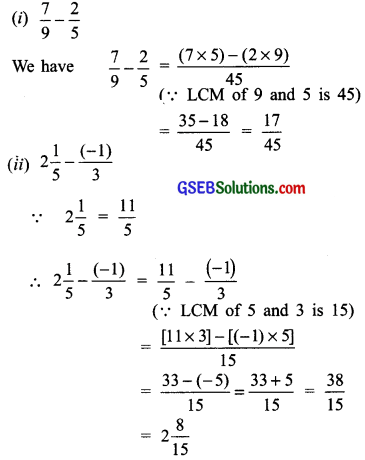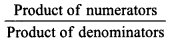# GSEB Solutions Class 7 Maths Chapter 9 Rational Numbers InText Questions

Gujarat Board GSEB Textbook Solutions Class 7 Maths Chapter 9 Rational Numbers InText Questions and Answers.

## Gujarat Board Textbook Solutions Class 7 Maths Chapter 9 Rational Numbers InText QuestionsTry These (Page 174)

Question 1.
Is the number $$\frac { 2 }{ -3 }$$ rational? Think about it.
Solution:
Yes, $$\frac { 2 }{ -3 }$$ is a rational number,
∵ 2 and – 3 are integers and – 3 ≠ 0.

Question 2.
List ten rational numbers.
Solution:
Following are ten rational numbers:
$$\frac { 1 }{ 3 }$$, $$\frac { 2 }{ -3 }$$, $$\frac { 4 }{ 5 }$$, $$\frac { 1 }{ -6 }$$, $$\frac { -3 }{ – 4 }$$ 5.8, 2$$\frac { 4 }{ 5 }$$, 0.93, 18 and 11.07.
Note.
1. ‘0’ can be written as $$\frac { 0 }{ 2 }$$ or $$\frac { 0 }{ 15 }$$, etc. Hence, it is a rational number.
2. A natural number can be written as 5 = $$\frac { 5 }{ 1 }$$ or 108
= $$\frac { 108 }{ 1 }$$
Hence, it is also a rational number.Try These (Page 175)

Question 1.
Fill in the boxes:Solution:Try These (Page 175)

Question 1.
Is 5 a positive rational number?
Solution:
Yes, 5 or $$\frac { 5 }{ 1 }$$ is having both its numerator and denominator as positive.
∴ It is a positive rational number.

Question 2.
List five more positive rational numbers.
Solution:
$$\frac { 1 }{ 7 }$$, $$\frac { 3 }{ 8 }$$, $$\frac { 5 }{ 17 }$$, $$\frac { 2 }{ 9 }$$ and $$\frac { 5 }{ 18 }$$ are positive rational numbers.Try These (Page 176)

Question 1.
Is – 8 a negative rational number?
Solution:
Yes, – 8 or $$\frac { -8 }{ 1 }$$ is a negative rational number, because its numerator is a negative integer.

Question 2.
List five more negative rational numbers.
Solution:
Five negative rational numbers are as follows:
$$\frac { – 5 }{ 9 }$$, $$\frac { -6 }{ 11 }$$, $$\frac { -3 }{ 13 }$$, $$\frac { 3 }{ -10 }$$ and $$\frac { -1 }{ 7 }$$

Try These (Page 176)

Question 1.
Which of these are negative rational numbers?
(i) $$\frac { -2 }{ 3 }$$
(ii) $$\frac { 5 }{ 7 }$$
(iii) $$\frac { 3 }{ -5 }$$
(iv) 0
(v) $$\frac { 6 }{ 11 }$$
(vi) $$\frac { -2 }{ -9 }$$
Solution:
(i) $$\frac { -2 }{ 3 }$$ is a negative rational number.
(ii) $$\frac { 5 }{ 7 }$$ is a positive rational number.
(iii) $$\frac { 3 }{ -5 }$$ is a negative rational number.
(iv) 0 is neither a positive nor a negative rational number.
(v) $$\frac { 6 }{ 11 }$$ is a positive rational number.
(vi) $$\frac { -2 }{ -9 }$$ is a positive rational number.

∴ (i) $$\frac { -2 }{ 3 }$$ and (ii) $$\frac { 3 }{ -5 }$$ are negative rational numbers.

Try These (Page 178)

Question 1.
Find the standard form of:
(i) $$\frac { -18 }{ 45 }$$
(ii) $$\frac { -12 }{ 18 }$$
Solution:
(i) Since HCF of 18 and 45 is 9.
∴ $$\frac { -18 }{ 45 }$$ = $$\frac { (-18)÷9 }{ 45÷9 }$$ = $$\frac { -2 }{ 5 }$$
Thus, the standard form of is $$\frac { -18 }{ 45 }$$ is $$\frac { -2 }{ 5 }$$

(ii) Since, HCF of 12 and 18 is 6.
∴ $$\frac { -12 }{ 18 }$$ = $$\frac { (-12)÷6 }{ 18÷6 }$$ = $$\frac { -2 }{ 3 }$$
Thus, the standard form of $$\frac { -12 }{ 18 }$$ is $$\frac { -2 }{ 3 }$$Try These (Page 181)

Question 1.
Find five rational numbers between $$\frac { -5 }{ 7 }$$ and $$\frac { -3 }{ 8 }$$.
Solution:
First we convert the given rational numbers with common denominators.
∵ LCM of 7 and 8 is 56.Thus, the five rational numbers, between $$\frac { -5 }{ 7 }$$ and $$\frac { -3 }{ 8 }$$ are:
$$\frac { -39 }{ 56 }$$, $$\frac { -38 }{ 56 }$$, $$\frac { -37 }{ 56 }$$, $$\frac { -36 }{ 56 }$$, $$\frac { -35 }{ 56 }$$
or $$\frac { -39 }{ 56 }$$, $$\frac { -19 }{ 28 }$$, $$\frac { -37 }{ 56 }$$, $$\frac { -9 }{ 14 }$$, $$\frac { -5 }{ 8 }$$

Try These (Page 185)

Question 1.
Find:
(i) $$\frac { -13 }{ 7 }$$ + $$\frac { 6 }{ 7 }$$
(ii) $$\frac { 19 }{ 5 }$$ + $$\frac { -7 }{ 5 }$$
Solution:Question 2.
Find:
(i) $$\frac { -3 }{ 7 }$$ + $$\frac { 2 }{ 3 }$$
(ii) $$\frac { -5 }{ 6 }$$ + $$\frac { -3 }{ 11 }$$
Solution:
(i) $$\frac { -3 }{ 7 }$$ + $$\frac { 2 }{ 3 }$$
∵ LCM of 7 and 3 is 21.
∴ $$\frac { -3 }{ 7 }$$ = $$\frac { (-3)×3 }{ 7×3 }$$ = $$\frac { -9 }{ 21 }$$
and $$\frac { 2 }{ 3 }$$ = $$\frac { 2×7 }{ 3×7 }$$ = $$\frac { 14 }{ 21 }$$
∴ $$\frac { -3 }{ 7 }$$ + $$\frac { 2 }{ 3 }$$ = $$\frac { -9 }{ 21 }$$ + $$\frac { 14 }{ 21 }$$
= $$\frac { -9+14 }{ 21 }$$
= $$\frac { 5 }{ 21 }$$

(ii) $$\frac { -5 }{ 6 }$$ + $$\frac { -3 }{ 11 }$$
Since, LCM of 6 and 11 is 66.Try These (Page 186)

Question 1.
What will be the additive inverse of $$\frac { -3 }{ 9 }$$? $$\frac { -9 }{ 11 }$$?$$\frac { 5 }{ 7 }$$?
Solution:
Additive inverse of $$\frac { -3 }{ 9 }$$ is $$\frac { 3 }{ 9 }$$
Additive inverse of $$\frac { -9 }{ 11 }$$ is $$\frac { 9 }{ 11 }$$
Additive inverse of $$\frac { 5 }{ 7 }$$ is $$\frac { -5 }{ 7 }$$Try These (Page 187)

Question 1.
Find:
(i) $$\frac { 7 }{ 9 }$$ – $$\frac { 2 }{ 5 }$$
(ii) 2$$\frac { 1 }{ 5 }$$ – $$\frac { -1 }{ 3 }$$
Solution:Try These (Page 188)

Question 1.
What will be
(i) $$\frac { -3 }{ 5 }$$ x 7?
(ii) $$\frac { -6 }{ 5 }$$ x (-2)?
Solution:
(i) $$\frac { -3 }{ 5 }$$ x 7 = $$\frac { (-3)×7 }{ 5 }$$ = $$\frac { -21 }{ 5 }$$
(ii) $$\frac { -6 }{ 5 }$$ x (-2) = $$\frac { -6×(-2) }{ 5 }$$ = $$\frac { 12 }{ 5 }$$

Note:
We multiply two rational numbers in the following way:
(i) Multiply the numerators of the rational numbers.
(ii) Multiply the denominators of the rational numbers.
(iii) Then product =For example:Try These (Page 188)

Question 1.
Find:
(i) $$\frac { -3 }{ 4 }$$ x $$\frac { 1 }{ 7 }$$
(ii) $$\frac { 2 }{ 3 }$$ x $$\frac { -5 }{ 9 }$$
Solution:Try These (Page 189)

Question 1.
What will be the reciprocal of $$\frac { -6 }{ 11 }$$ and $$\frac { -8 }{ 5 }$$?
Solution:
(i) Reciprocal of $$\frac { -6 }{ 11 }$$ is $$\frac { 11 }{ -6 }$$
(ii) Reciprocal of $$\frac { -8 }{ 5 }$$ is $$\frac { -5 }{ 8 }$$.
To divide one rational number by the other rational number, we multiply the rational number by the reciprocal of the other. For example,Try These (Page 190)

Question 1.
Find:
(i) $$\frac { 2 }{ 3 }$$ x $$\frac { -7 }{ 8 }$$
(ii) $$\frac { -6 }{ 7 }$$ x $$\frac { 5 }{ 7 }$$
Solution: